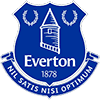2022 EPL Top Goalscorer of the Year Odds
-2500
0.04 to 1Erling Haaland
71.0% implied probability

+1400
14 to 1Harry Kane
4.9% implied probability

+5000
50 to 1Marcus Rashford
1.4% implied probability

+6500
65 to 1Timo Werner
1.1% implied probability

+10000
100 to 1Cristiano Ronaldo
0.7% implied probability

+10000
100 to 1Darwin Gabriel Nunez Ribeiro
0.7% implied probability

+10000
100 to 1Luis Diaz
0.7% implied probability

+10000
100 to 1Gianluca Scamacca
0.7% implied probability

+10000
100 to 1Ivan Toney
0.7% implied probability

+10000
100 to 1Jarrod Bowen
0.7% implied probability

+15000
150 to 1Callum Wilson
0.5% implied probability

+15000
150 to 1Mohamed Salah
0.5% implied probability

+15000
150 to 1Eddie Nketiah
0.5% implied probability

+15000
150 to 1Dominic Solanke
0.5% implied probability

+20000
200 to 1Taiwo Awoniyi
0.4% implied probability

+20000
200 to 1Michail Antonio
0.4% implied probability

+20000
200 to 1Che Adams
0.4% implied probability

+20000
200 to 1Kevin De Bruyne
0.4% implied probability

+20000
200 to 1Bukayo Saka
0.4% implied probability

+20000
200 to 1Brennan Johnson
0.4% implied probability

+20000
200 to 1Ollie Watkins
0.4% implied probability

+20000
200 to 1Neal Maupay
0.4% implied probability

+20000
200 to 1Jesse Lingard
0.4% implied probability

+20000
200 to 1Kelechi Iheanacho
0.4% implied probability

+20000
200 to 1Patrick Bamford
0.4% implied probability

+20000
200 to 1Aleksandar Mitrovic
0.4% implied probability

+20000
200 to 1Leandro Trossard
0.4% implied probability

+20000
200 to 1Raul Jimenez
0.4% implied probability

+20000
200 to 1Danny Ings
0.4% implied probability

+20000
200 to 1Riyad Mahrez
0.4% implied probability

+25000
250 to 1Gabriel Martinelli
0.3% implied probability

+25000
250 to 1Emile Smith Rowe
0.3% implied probability

+25000
250 to 1Brenden Aaronson
0.3% implied probability

+25000
250 to 1Antony Matheus dos Santos
0.3% implied probability

+25000
250 to 1Pierre-Emerick Aubameyang
0.3% implied probability

+25000
250 to 1Patson Daka
0.3% implied probability

+25000
250 to 1Deniz Undav
0.3% implied probability

+25000
250 to 1Philippe Coutinho
0.3% implied probability

+25000
250 to 1Julian Alvarez
0.3% implied probability

+25000
250 to 1Alexander Isak
0.3% implied probability

+25000
250 to 1Heung-Min Son
0.3% implied probability

+25000
250 to 1Bruno Guimaraes Rodriguez Moura
0.3% implied probability

+25000
250 to 1Mason Mount
0.3% implied probability

+25000
250 to 1Raheem Sterling
0.3% implied probability

+25000
250 to 1Jamie Vardy
0.3% implied probability

+25000
250 to 1Bernardo Silva
0.3% implied probability

+25000
250 to 1Anthony Martial
0.3% implied probability

+25000
250 to 1Wilfried Zaha
0.3% implied probability

+25000
250 to 1Christian Pulisic
0.3% implied probability

+25000
250 to 1Bruno Miguel Borges Fernandes
0.3% implied probability

+25000
250 to 1Diogo Jota
0.3% implied probability

+25000
250 to 1Danny Welbeck
0.3% implied probability

+25000
250 to 1Kai Havertz
0.3% implied probability

+25000
250 to 1Dominic Calvert-Lewin
0.3% implied probability

+25000
250 to 1Gabriel Jesus
0.3% implied probability

+25000
250 to 1Richarlison De Andrade
0.3% implied probability

+25000
250 to 1Phil Foden
0.3% implied probability

+25000
250 to 1Jadon Sancho
0.3% implied probability

+25000
250 to 1James Maddison
0.3% implied probability

+50000
500 to 1Luis Fernando Sinisterra Lucumi
0.1% implied probability

+50000
500 to 1Roberto Firmino
0.1% implied probability

+50000
500 to 1Chris Wood
0.1% implied probability

+50000
500 to 1Rodrigo Moreno Machado
0.1% implied probability

+50000
500 to 1Jack Grealish
0.1% implied probability